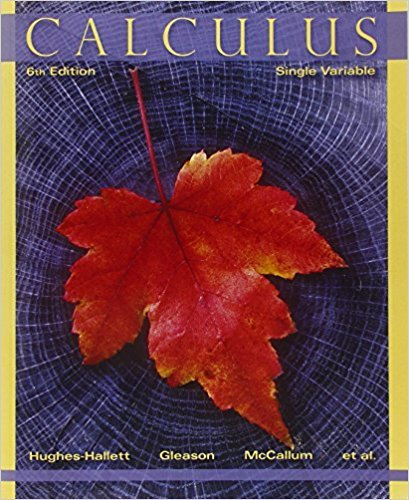×
×

# Solutions for Chapter 7.7: COMPARISON OF IMPROPER INTEGRALS## Full solutions for Calculus: Single Variable | 6th Edition

ISBN: 9780470888643Solutions for Chapter 7.7: COMPARISON OF IMPROPER INTEGRALS

Solutions for Chapter 7.7
4 5 0 390 Reviews
31
4
##### ISBN: 9780470888643

Calculus: Single Variable was written by and is associated to the ISBN: 9780470888643. Since 39 problems in chapter 7.7: COMPARISON OF IMPROPER INTEGRALS have been answered, more than 33501 students have viewed full step-by-step solutions from this chapter. This textbook survival guide was created for the textbook: Calculus: Single Variable , edition: 6. Chapter 7.7: COMPARISON OF IMPROPER INTEGRALS includes 39 full step-by-step solutions. This expansive textbook survival guide covers the following chapters and their solutions.

Key Calculus Terms and definitions covered in this textbook
• Absolute minimum

A value ƒ(c) is an absolute minimum value of ƒ if ƒ(c) ? ƒ(x)for all x in the domain of ƒ.

• Additive inverse of a complex number

The opposite of a + bi, or -a - bi

• Arcsecant function

See Inverse secant function.

• Census

An observational study that gathers data from an entire population

• Directed line segment

See Arrow.

• Equivalent vectors

Vectors with the same magnitude and direction.

• Grapher or graphing utility

Graphing calculator or a computer with graphing software.

• Hypotenuse

Side opposite the right angle in a right triangle.

• Independent events

Events A and B such that P(A and B) = P(A)P(B)

• Inequality symbol or

<,>,<,>.

• Logarithmic form

An equation written with logarithms instead of exponents

• Logarithmic regression

See Natural logarithmic regression

• Number line graph of a linear inequality

The graph of the solutions of a linear inequality (in x) on a number line

• Orthogonal vectors

Two vectors u and v with u x v = 0.

• Polynomial function

A function in which ƒ(x)is a polynomial in x, p. 158.

• Random behavior

Behavior that is determined only by the laws of probability.

• Random variable

A function that assigns real-number values to the outcomes in a sample space.

• Sequence

See Finite sequence, Infinite sequence.

• Standard form: equation of a circle

(x - h)2 + (y - k2) = r 2

• Symmetric matrix

A matrix A = [aij] with the property aij = aji for all i and j

×